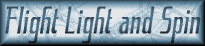Oscillations in Rotation Curves of Galaxies When the rotation curve line of spiral galaxies oscillates (sometimes faster and sometimes slower) with distance like a sin curve, then the gravitational pair exhibits a more irregular orbital pattern. In the image below the distance between the binary pair varies. This explains why some of the curves shown in the given question oscillate, and other lines are uniform. When the orbits of the pair overlap in neat circles then the velocities of the stars remain constant and there is no oscillation. If the orbits intersect, then the distance between them varies. The velocities of the stars emitted then spiral outwards with the same variation in velocity.In the simulation above, the orbital pair have a vastly exaggerated and irregular orbit so the oscillation is extreme. Some stars rotate outwards at vastly different velocities to others. If the orbital pair of super-massive bodies move way from each other, then the stars they emit rotate outwards more slowly. When the orbital pair are close together, then the stars rotate outwards at higher velocities. As the orbits of the super-massive bodies oscillate, so the velocity of the stars' orbits oscillate.

 Click the link below and Download Orbit Game 4.3 (August 2014) >> orbit-game-4-3.exe << orbit-game-4-3.exe demonstrates how the binary pair move away from each other as they lose mass due to stars having being emitted. Thus stars emitted subsequently are effected by a lesser gravitational force, moving them more slowly. The outer and older stars have often reached escape velocity. If your PC is set to use the comma as a decimal separator, then these apps won't work so good.

 In the system below the white holes are perfectly balanced. Thus the stars’ velocities reflect uniformity; and no oscillation occurs.

 Latest Article: Gravitational Waves & General Relativity A computational analysis of the theory of gravitational waves; as expressed within Einstein’s Theory of General Relativity and the wider realm of Astrophysics. Essentially a critique, this study has been written for the purpose of explaining the unobvious challenges faced in building graphically dynamic evolutionary computer models. These models compute the theoretical functionality of gravitational waves in the celestial paradigms of solar system formation and galaxy formation.

< top >

< seti >

< why? >

< credits >

< contact >

< dark matter >

< dark energy >

< binary orbits >

< the big unwind >

< force of gravity >

< centrifugal force >

< zeno and planck >

< quantum gravity >

< principle of flight >

< relativity revised >

< gravity simulators >

< 3d printer projects >

< entothopter videos >

< why is the sky blue? >

< free fractal software >

< entothopter project >

< gravitational lensing >

< sketchup entothopter >

< ezekiel's flying machine >

< the many-body problem >

< philosophy of computers >

< genealogy and exponents >

< bin laden helicopter crash >

< how the solar system formed >

< rubin's problem - introduction >

< rubin's problem solved in animation >

< gravitational waves & general relativity >

< why the moon is receding from the earth >

< why the moon is red during a lunar eclipse >